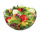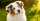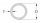# Fraction calculator

The calculator performs basic and advanced operations with fractions, expressions with fractions combined with integers, decimals, and mixed numbers. It also shows detailed step-by-step information about the fraction calculation procedure. Solve problems with two, three, or more fractions and numbers in one expression.

## Result:

### 32/5 - 3/4 + 51/2 = 163/20 = 8 3/20 = 8.15

Spelled result in words is one hundred sixty-three twentieths (or eight and three twentieths).

### How do you solve fractions step by step?

1. Conversion a mixed number 3 2/5 to a improper fraction: 3 2/5 = 3 2/5 = 3 · 5 + 2/5 = 15 + 2/5 = 17/5

To find new numerator:
a) Multiply the whole number 3 by the denominator 5. Whole number 3 equally 3 * 5/5 = 15/5
b) Add the answer from previous step 15 to the numerator 2. New numerator is 15 + 2 = 17
c) Write a previous answer (new numerator 17) over the denominator 5.

Three and two fifths is seventeen fifths
2. Subtract: 17/5 - 3/4 = 17 · 4/5 · 4 - 3 · 5/4 · 5 = 68/20 - 15/20 = 68 - 15/20 = 53/20
For adding, subtracting, and comparing fractions, it is suitable to adjust both fractions to a common (equal, identical) denominator. The common denominator you can calculate as the least common multiple of both denominators - LCM(5, 4) = 20. In practice, it is enough to find the common denominator (not necessarily the lowest) by multiplying the denominators: 5 × 4 = 20. In the next intermediate step, the fraction result cannot be further simplified by canceling.
In words - seventeen fifths minus three quarters = fifty-three twentieths.
3. Conversion a mixed number 5 1/2 to a improper fraction: 5 1/2 = 5 1/2 = 5 · 2 + 1/2 = 10 + 1/2 = 11/2

To find new numerator:
a) Multiply the whole number 5 by the denominator 2. Whole number 5 equally 5 * 2/2 = 10/2
b) Add the answer from previous step 10 to the numerator 1. New numerator is 10 + 1 = 11
c) Write a previous answer (new numerator 11) over the denominator 2.

Five and one half is eleven halfs
4. Add: the result of step No. 2 + 11/2 = 53/20 + 11/2 = 53/20 + 11 · 10/2 · 10 = 53/20 + 110/20 = 53 + 110/20 = 163/20
For adding, subtracting, and comparing fractions, it is suitable to adjust both fractions to a common (equal, identical) denominator. The common denominator you can calculate as the least common multiple of both denominators - LCM(20, 2) = 20. In practice, it is enough to find the common denominator (not necessarily the lowest) by multiplying the denominators: 20 × 2 = 40. In the next intermediate step, the fraction result cannot be further simplified by canceling.
In words - fifty-three twentieths plus eleven halfs = one hundred sixty-three twentieths.

#### Rules for expressions with fractions:

Fractions - use the slash “/” between the numerator and denominator, i.e., for five-hundredths, enter 5/100. If you are using mixed numbers, be sure to leave a single space between the whole and fraction part.
The slash separates the numerator (number above a fraction line) and denominator (number below).

Mixed numerals (mixed fractions or mixed numbers) write as non-zero integer separated by one space and fraction i.e., 1 2/3 (having the same sign). An example of a negative mixed fraction: -5 1/2.
Because slash is both signs for fraction line and division, we recommended use colon (:) as the operator of division fractions i.e., 1/2 : 3.

Decimals (decimal numbers) enter with a decimal point . and they are automatically converted to fractions - i.e. 1.45.

The colon : and slash / is the symbol of division. Can be used to divide mixed numbers 1 2/3 : 4 3/8 or can be used for write complex fractions i.e. 1/2 : 1/3.
An asterisk * or × is the symbol for multiplication.
Plus + is addition, minus sign - is subtraction and ()[] is mathematical parentheses.
The exponentiation/power symbol is ^ - for example: (7/8-4/5)^2 = (7/8-4/5)2

#### Examples:

subtracting fractions: 2/3 - 1/2
multiplying fractions: 7/8 * 3/9
dividing Fractions: 1/2 : 3/4
exponentiation of fraction: 3/5^3
fractional exponents: 16 ^ 1/2
adding fractions and mixed numbers: 8/5 + 6 2/7
dividing integer and fraction: 5 ÷ 1/2
complex fractions: 5/8 : 2 2/3
decimal to fraction: 0.625
Fraction to Decimal: 1/4
Fraction to Percent: 1/8 %
comparing fractions: 1/4 2/3
multiplying a fraction by a whole number: 6 * 3/4
square root of a fraction: sqrt(1/16)
reducing or simplifying the fraction (simplification) - dividing the numerator and denominator of a fraction by the same non-zero number - equivalent fraction: 4/22
expression with brackets: 1/3 * (1/2 - 3 3/8)
compound fraction: 3/4 of 5/7
fractions multiple: 2/3 of 3/5
divide to find the quotient: 3/5 ÷ 2/3

The calculator follows well-known rules for order of operations. The most common mnemonics for remembering this order of operations are:
PEMDAS - Parentheses, Exponents, Multiplication, Division, Addition, Subtraction.
BEDMAS - Brackets, Exponents, Division, Multiplication, Addition, Subtraction
BODMAS - Brackets, Of or Order, Division, Multiplication, Addition, Subtraction.
GEMDAS - Grouping Symbols - brackets (){}, Exponents, Multiplication, Division, Addition, Subtraction.
Be careful, always do multiplication and division before addition and subtraction. Some operators (+ and -) and (* and /) has the same priority and then must evaluate from left to right.

## Fractions in word problems:

• Circular gardenAlice creates a circular vegetable garden. Tomatoes are planted in 1/3 of the circular garden, carrots are planted in 2/5 of the circular garden, and green peppers are planted in 1/10 of the circular garden. What fraction represents the remaining unplante
• Berry SmoothieRory has 5/8 cup of milk. How much milk does she have left after she doubles the recipe of the smoothie? Berry Smoothie: 2 cups strawberries 1 cup blueberries 1/4 cup milk 1 tbsp (tablespoon) sugar 1/2 tsp (teaspoon) lemon juice 1/8 tsp (teaspoon) vanilla
• Sum of 18Sum of two fractions is 4 3/7. If one of the fractions is 2 1/5 find the other one .We need two tenths kg of a carrot, one tenth of peas and three tenths of of tomatoes to make salad. Express the fraction of the weight of the vegetables to be salad. Convert the result to grams.
• BaxterBaxter ate 1/12 of a box of dog food. Now the box is 3/4 full. What fraction of the full of a full box was there before Baxter?
• Two pizzasJacobs mom bought two whole pizzas. He ate 2/10 of the pizza and his dad ate 1 1/5. How much is left.
• The book 4Mr. Kinion read 3 3/4 chapters in his book on Monday. He then read 2 4/6 more chapters on Tuesday. How many chapters has he read so far?Why does 1 3/4 + 2 9/10 equal 4.65? How do you solve this?Skilled workshop master washes client car 1/5 hour, cleaned the client's car in 5/4 hour, and painted small defects on car 1 1/3 hour. How long did it take him to do all the necessary work tasks?If the quotient of [8/5 divided by 8/10] is added to the product of [8/14 x 7/12 x 3/8], what is the sum?Eight pipes are each 2¼m long . what is the total length of the eight pipes?Evaluate 2x+6y when x=- 4/5 and y=1/3. Write your answer as a fraction or mixed number in simplest form.To three-eighths of one third, we add five quarters of one half and multiply the sum by four. How much will we get?